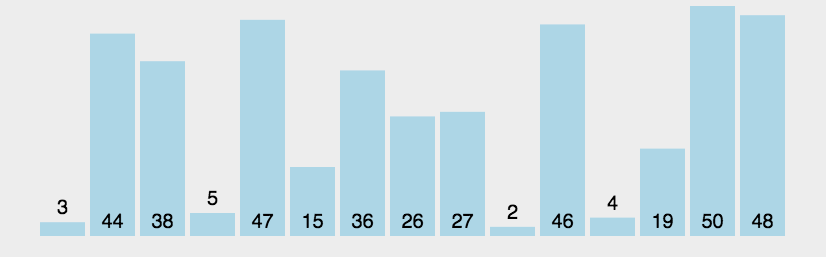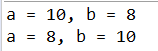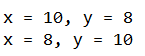# 二、算法思想

...# 三、代码实现

for (int i = 0; i < arr.length - 1; i++) {
for (int j = 0; j < arr.length - 1 - i; j++) {
if (arr[j] > arr[j + 1]) {
SortUtil.swap(arr, j, j + 1);
}
}
}

# 四、数字换位的三种方法

## 4.1 加减换位法

/* 1、加减换位法*/
int a = 10;
int b = 8;
System.out.println("a = " + a + ", b = " + b);
a = a + b; // 18
b = a - b; // 10
a = a - b; // 8
System.out.println("a = " + a + ", b = " + b);## 4.2 异或换位法

/* 2、异或换位法*/
int x = 10;
int y = 8;
System.out.println("x = " + x + ", y = " + y);
x = x ^ y;
y = x ^ y;
x = x ^ y;
System.out.println("x = " + x + ", y = " + y);public static void main(String[] args) {
int[] arr = {3, 2, 1, 4};
SortUtil.printArr(arr);
int idx = 1;
arr[idx] = arr[idx] ^ arr[idx];
arr[idx] = arr[idx] ^ arr[idx];
arr[idx] = arr[idx] ^ arr[idx];
SortUtil.printArr(arr);
}
3	2	1	4
3	0	1	4	

## 4.3 异或运算

x = 10 = 1010B

y = 8 = 1000B

① x = x ^ y = 1010 ^ 1000 = 0010

② y = x ^ y = 0010 ^ 1000 = 1010 = 10

③ x = x ^ y = 0010 ^ 1010 = 1000 = 8

// 规定：相异为0， 相同为 1

x = x ^ y = 1010 ^ 1000 = 1101

y = x ^ y = 1101 ^ 1000 = 1010 = 10

x = x ^ y = 1101 ^ 1010 = 1000 = 8

07-0601-247050
05-059216
07-116632
08-082042
12-2668
09-2057
10-31608
08-162万+
04-246864
01-121117
05-14
07-238897
04-067092
09-1847
08-293919
06-043204
02-251321
05-24